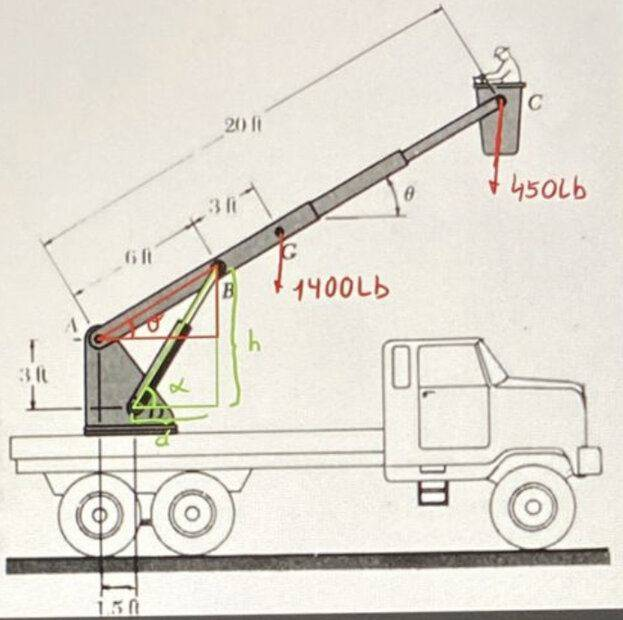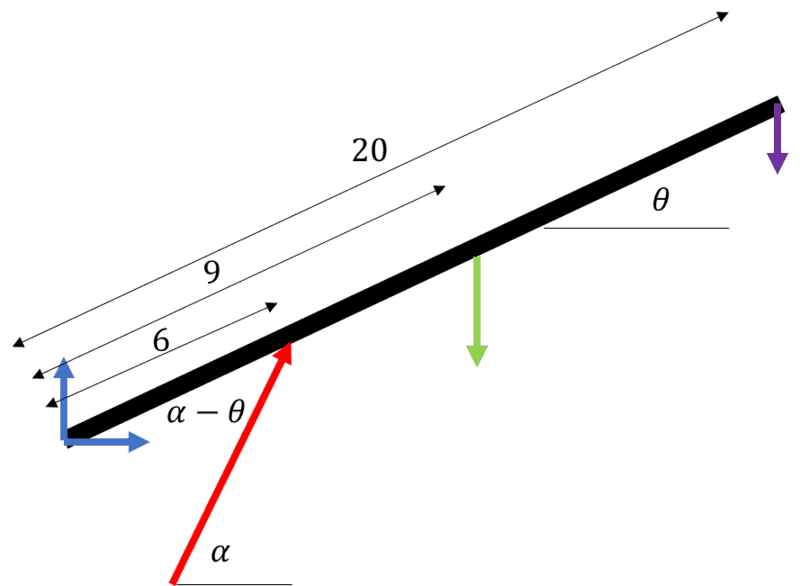# Calculating ##F_{DB}##: Equilibrium in a Machine

• Guillem_dlc
In summary, Guillem_dlc argues that the two force components should be applied at D, whereas the OP argues they should be applied at B. While the two force components may be equivalent algebraically, the OP's argument for applying them at B is more convincing.f

#### Guillem_dlc

Homework Statement
The ABC telescopic extension arm is used to lift a worker to the height of overhead, telephone and electrical cables. For the indicated extension, the centre of gravity of the ##1400\, \textrm{lb}##-arm is at the ##G##-point. The worker, the basket and the fixed equipment in the basket weigh ##450\, \textrm{lb}## and their combined centre of gravity is at point ##C##. For the given position, determine the force exerted at ##B## by the single hydraulic jack using when ##\theta =35\, \textrm{º}##.

Solution: ##\vec{F}_{DB}=6490\, \textrm{lb}\,\,\, 62,1\, \textrm{º}##
Relevant Equations
##\sum M=0##
Figure:My attempt at a solution:
For the calculation of ##F_{DB}## we consider the equilibrium in the whole machine.
$$\left. \begin{array}{r} h\rightarrow h=6\cdot \sin \theta +3=6,44\, \textrm{ft} \\ d\rightarrow d=6\cos \theta -1,5=3,41\, \textrm{ft} \end{array}\right\} \,\, \alpha =\arctan \left( \dfrac{h}{d}\right) =62,1\, \textrm{º}$$
$$\sum M_A=0\rightarrow -9\cos \theta G-20\cos \theta C+F_{DB}\cos \alpha \cdot 3+F_{DB}\sin \alpha \cdot 1,6$$
$$\rightarrow \boxed{F_{DB}=6482,5\, \textrm{lb}}$$
This one is well done, isn't it? It doesn't give me the exact toto but I guess it will be fine.

Homework Statement:: The ABC telescopic extension arm is used to lift a worker to the height of overhead, telephone and electrical cables. For the indicated extension, the centre of gravity of the ##1400\, \textrm{lb}##-arm is at the ##G##-point. The worker, the basket and the fixed equipment in the basket weigh ##450\, \textrm{lb}## and their combined centre of gravity is at point ##C##. For the given position, determine the force exerted at ##B## by the single hydraulic jack using when ##\theta =35\, \textrm{º}##.

Solution: ##\vec{F}_{DB}=6490\, \textrm{lb}\,\,\, 62,1\, \textrm{º}##
Relevant Equations:: ##\sum M=0##

Figure:
View attachment 316600
My attempt at a solution:
For the calculation of ##F_{DB}## we consider the equilibrium in the whole machine.
$$\left. \begin{array}{r} h\rightarrow h=6\cdot \sin \theta +3=6,44\, \textrm{ft} \\ d\rightarrow d=6\cos \theta -1,5=3,41\, \textrm{ft} \end{array}\right\} \,\, \alpha =\arctan \left( \dfrac{h}{d}\right) =62,1\, \textrm{º}$$
$$\sum M_A=0\rightarrow -9\cos \theta G-20\cos \theta C+F_{DB}\cos \alpha \cdot 3+F_{DB}\sin \alpha \cdot 1,6$$
$$\rightarrow \boxed{F_{DB}=6482,5\, \textrm{lb}}$$
This one is well done, isn't it? It doesn't give me the exact toto but I guess it will be fine.
I hope I'm not stepping out of line, but I don't believe PF stives to be a homework checking service. Did you have a specific concern about the problem?

I hope I'm not stepping out of line, but I don't believe PF stives to be a homework checking service. Did you have a specific concern about the problem?
I just wanted to make sure I got it right, because I only have the solution. Sorry for the inconvenience.

We'll if you got that close to the official answer, it's probably either some rounding error carried through computation or sig fig consideration. You are within 0.1% of the book result.

•Guillem_dlc
$$\sum M_A=0\rightarrow -9\cos \theta G-20\cos \theta C+F_{DB}\cos \alpha \cdot 3+F_{DB}\sin \alpha \cdot 1,6$$
I don't understand the lever arm length 1,6 used on the right. Shouldn't it be 1,5? Maybe a typo when posting.

For the answer, I get 6480, almost exactly.

Last edited:
Well, go figure. I get something different from everyone (and the book)

$$\circlearrowleft^+ \sum M_A = 0 = -9 G \cos \theta -20 C \cos \theta + 6 F \sin ( \alpha - \theta)$$

##F \approx 6473 \rm{lb}##

Last edited:
Well, go figure. I get something different from everyone (and the book)

$$\circlearrowleft^+ \sum M_A = 0 = -9 G \cos \theta -20 C \cos \theta + 6 F \sin ( \alpha - \theta)$$

##F \approx 6473 N##
Again, the discrepancy looks like rounding error. Yours is only 0.1% below mine (if you change N to lb).

Again, the discrepancy looks like rounding error. Yours is only 0.1% below mine (if you change N to lb).
I don't know, something seems fishy.

Taking ##6473 \rm{lb}## as ##6490 \rm{lb}## seem like a bit of a stretch for rounding error. I used stored values for ##\alpha##, and rounded value for ##\alpha ## and I get ## \approx 6474 \rm{lb}##.

Everything else is a single computation with exact values.

Looking carefully at what the OP did, neither of the last two term makes sense to me.

This is the free body diagram of the arm.Edit: I think I see the intention of the OP now, but if everything is equivalent why the noticeably different results?

Last edited:
Looking carefully at what the OP did, neither of the last two term makes sense to me.

This is the free body diagram of the arm.

View attachment 316628
@Guillem_dlc is taking the two force components as being applied at D, though there may be a small error in the arithmetic; see post #5. I took them as applied at B, which gives a different combination of lever arms but should be equivalent. My form is readily converted to yours, so I think all three algebras are fine.

•erobz
@Guillem_dlc is taking the two force components as being applied at D, though there may be a small error in the arithmetic; see post #5. I took them as applied at B, which gives a different combination of lever arms but should be equivalent. My form is readily converted to yours, so I think all three algebras are fine.
I’m still perplexed by the significance of computational error between ours if you say they are equivalent algebraically? Having a 0.1% error between it an the book is one thing. Having a 0.1% error between two equivalent methods is something else…It’s late, maybe I’m tired and goofing up somehow.

$$\circlearrowleft^+ \sum M_A = 0 = -9 G \cos \theta -20 C \cos \theta + 6 F \sin ( \alpha - \theta)$$

##F \approx 6473 \rm{lb}##
$$\sum M_A=0\rightarrow -9\cos \theta G-20\cos \theta C+F_{DB}\cos \alpha \cdot 3+F_{DB}\sin \alpha \cdot 1,5$$
$$\rightarrow \boxed{F_{DB}=6482,5\, \textrm{lb}}$$

So as far as I can see, there are at least ( @haruspex has a third equivalent) three ways of approaching it that should be equivalent. All three should yield the same result. Yet all three give very noticeably different results from each other (none giving the book result)! There has to be something interesting here that explains this. If it was a difference in decimal places, I'd be un-surprised...but a difference of ##10 \, \rm{lb}## between them?

•Lnewqban
So as far as I can see, there are at least ( @haruspex has a third equivalent) three ways of approaching it that should be equivalent. All three should yield the same result. Yet all three give very noticeably different results from each other (none giving the book result)! There has to be something interesting here that explains this. If it was a difference in decimal places, I'd be un-surprised...but a difference of ##10 \, \rm{lb}## between them?
The most likely source of rounding error would be in finding alpha then plugging that by hand into trig functions. If you manage to do everything in a single equation typed into a calculator it should keep plenty of digits.
Note that it is not necessary to find alpha as such since the sin and cos can be derived algebraically from tan.

I have now put all my working into a spreadsheet, which keeps 10 or so significant figs, it seems. I get 6480.106…
If we can agree on 6480 then my guess would be that the book's 6490 is a typo.

Are you using a spreadsheet or equivalent? If doing that you still get 6473 please post your values for alpha and its trig functions. I get 1.083323924 radians, cos: 0.4683942052, tan: 1.886273531.

•erobz
The most likely source of rounding error would be in finding alpha then plugging that by hand into trig functions. If you manage to do everything in a single equation typed into a calculator it should keep plenty of digits.
Note that it is not necessary to find alpha as such since the sin and cos can be derived algebraically from tan.

I have now put all my working into a spreadsheet, which keeps 10 or so significant figs, it seems. I get 6480.106…
If we can agree on 6480 then my guess would be that the book's 6490 is a typo.

Are you using a spreadsheet or equivalent? If doing that you still get 6473 please post your values for alpha and its trig functions. I get 1.083323924 radians, cos: 0.4683942052, tan: 1.886273531.
I get the same thing as you when I use Mathcad. I was using a TI-89, I think the rounding on the ##h## and ##d## that I just pulled from the OP was the culprit. I got 6480 on both now. Mamma mia!•haruspex